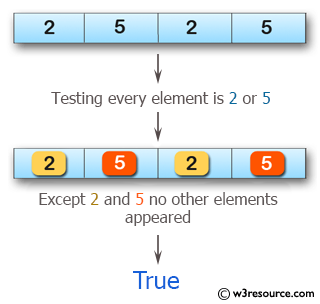﻿ Swift Array Programming Exercise: Test if every element is a 2 or a 5 of a given array of integers - w3resource# Swift Array Programming Exercises: Test if every element is a 2 or a 5 of a given array of integers

## Swift Array Programming: Exercise-29 with Solution

Write a Swift program to test if every element is a 2 or a 5 of a given array of integers.

Pictorial Presentation:Sample Solution:

Swift Code:

``````func num15(array_nums: [Int]) -> Bool {
for x in 0..<array_nums.count
{
if array_nums[x] != 2 && array_nums[x] != 5
{
return false
}
}
return true
}

print(num15(array_nums: [1, 2, 5, 4]))
print(num15(array_nums: [2, 5, 2, 5]))
print(num15(array_nums: [2, 5]))
```
```

Sample Output:

```false
true
true
```

Swift Programming Code Editor:

Improve this sample solution and post your code through Disqus

What is the difficulty level of this exercise?

﻿